## Chaos kernel function using the logistic map?

### Chaos kernel function using the logistic map?

Last updated 2858 days ago by JohnLast Comments (20)

I made some experiments with different kernels and I would like to share among friends some ideas.

Well it is about to use a function expressing chaotic behaviour itself as a kernel.

The simplest thing was to look at the most simple logistic map.That is the equation of the logistic map. What we can do with that.

What is interesting for us is the parameter r. If we vary the parameter we will have different behaviour (sensitivity of the initial conditions). I keep it short here but we can get some very interesting  stretching-and-folding structures , please look at the Wikipedia article.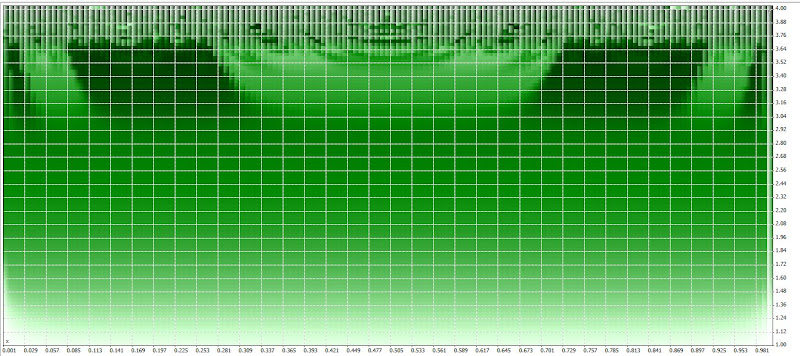От Поле за пускане

#property version "1.00"
//--- input parameters
input double x=0.5;

input double r=1;
//+------------------------------------------------------------------+
//| Tester function |
//+------------------------------------------------------------------+
double OnTester()
{
//---

double w0 = r*x*(1-x);
double w1 = r*w0*(1-w0);
double w2 = r*w1*(1-w1);
double w3 = r*w2*(1-w2);
double w4 = r*w3*(1-w3);
//---
return(w2);
}

This is the code that can be used to make mathematical simulations in Metatrader 5.

Here I do not use for and while operators, because I want the code to be mathematically explicit for the non coders here.

Well what we can do next and yes we can replace the x by the gaussian kernel (the gaussian kernet iteslf has x and y and the output varies from 0 to 1). So it is fine let take a look what we get.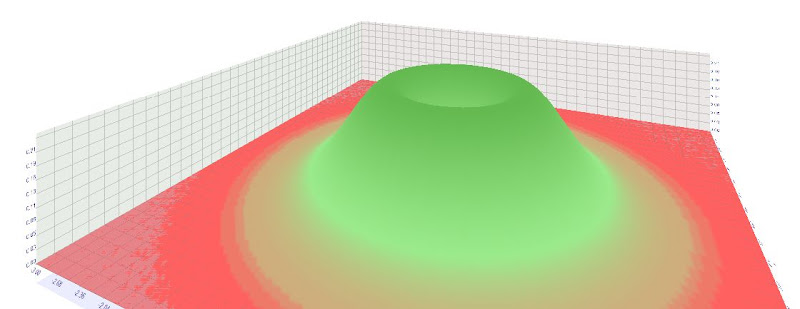От Поле за пускане

The code is here:

#property version "1.00"
//--- input parameters
input double x=-3.0;
input double y=-3.0;
input double r=1;
//+------------------------------------------------------------------+
//| Tester function |
//+------------------------------------------------------------------+
double OnTester()
{
//---

double w0 = MathExp(-x*x-y*y);
double w1 = r*w0*(1-w0);
double w2 = r*w1*(1-w1);
double w3 = r*w2*(1-w2);
double w4 = r*w3*(1-w3);
//---
return(w2);

So in the chaos kernel we have 3 parameters (look at the comments). x and y are coming from the gaussian kernel the r is from the logistic map equation.

But there is something more, lol, look at the recurrence relation, we can use the first cycle w1, the second, w2, the thirs w3 (it is up to us to choose how far we can go). So w2 is more complex that w0 and w3 has a more complex structure than w2.

The logistic map stretching-and-folding structures combined with an input coming gaussian kernel are able to produce weird things lol.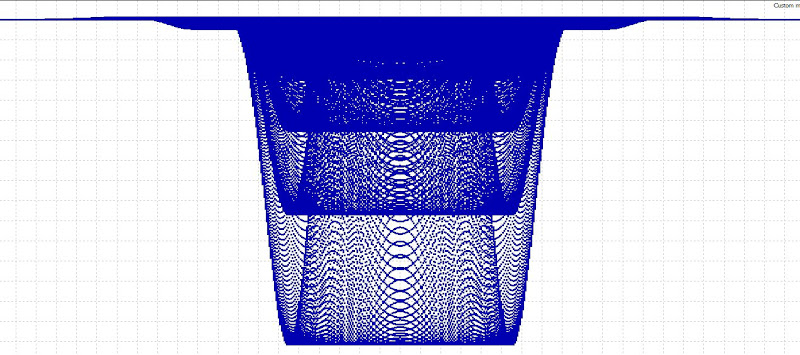От Поле за пускане

So can we use this as a kernel? The r parameter is the kernel's chaotic parameter than can be limited or expanded.

In my imagination the real science fiction artificial intelligence need to use some kind of chaotic parameter, stepping from the deterministic to the non deterministic.

The question is:

Can we use kernels with chaotic behavior to model chaotic systems?

I think yes too, if that is true that is another kernel trick.

• JohnLast 2876 days ago

The chaotic kernel looks like this. You can use w1, w2,w3 or w4 etc. In the example here we use w3.

For x1,x2,x3,x4 I use from -3 to 3 with step size 0.01

For r I use from 1 to 3.54, but you can decide how chaotic the kernel will be.

double chaos kernel()
{
double w0 = MathExp(gamma*(-x1*x1-y1*y1));
double w1 = r1*w0*(1-w0);
double w2 = r1*w1*(1-w1);
double w3= r1*w2*(1-w2);
double w4= r1*w3*(1-w3);
double w5= r1*w4*(1-w4);

double p1 = iCustom(TimeFrame,Symbol(),"PFE",x5,true,5,0,0);
double p2 = iCustom(TimeFrame,Symbol(),"PFE",x6,true,5,0,0);
double p3 = iCustom(TimeFrame,Symbol(),"PFE",x7,true,5,0,0);
double p4 = iCustom(TimeFrame,Symbol(),"PFE",x8,true,5,0,0);

return(w3* p1 + w3* p2 + w3*p3 + w3* p4);
}

• jaguar1637 2876 days ago

Phenomenal

So, this is the true kernel perceptron. very good.

Why in MQL5 and not in MQL4 ?

• jaguar1637 2876 days ago

and what is the value for Gamma ??

• jaguar1637 2876 days ago

The problem is how to fetch the appropriate value for r, too

you wrote "For r I use from 1 to 3.54" . Where r is defined ? in which equation ? which are the relationship between r and gamma ?

• JohnLast 2876 days ago

It is in mt5 because you can plot the mathematical function, so you can make visualisations very simply.

r is defined in the wikipewdia article. If the r is low there is no chaos, if you increase it above certain levels you get chaotic.

gamma is not needed, this is a mod.

## Behavior dependent on r

By varying the parameter r, the following behavior is observed:

• With r between 0 and 1, the population will eventually die, independent of the initial population.
• With r between 1 and 2, the population will quickly approach the value, independent of the initial population.
• With r between 2 and 3, the population will also eventually approach the same value, but first will fluctuate around that value for some time. The rate of convergence is linear, except for r=3, when it is dramatically slow, less than linear.
• With r between 3 and(approximately 3.45), from almost all initial conditions the population will approach permanent oscillations between two values. These two values are dependent on r.
• With r between 3.45 and 3.54 (approximately), from almost all initial conditions the population will approach permanent oscillations among four values.
• With r increasing beyond 3.54, from almost all initial conditions the population will approach oscillations among 8 values, then 16, 32, etc. The lengths of the parameter intervals which yield oscillations of a givenlength decrease rapidly; the ratio between the lengths of two successive such bifurcation intervals approaches the Feigenbaum constant δ = 4.669. This behavior is an example of a period-doubling cascade.
• At r approximately 3.57 is the onset of chaos, at the end of the period-doubling cascade. From almost all initial conditions we can no longer see any oscillations of finite period. Slight variations in the initial population yield dramatically different results over time, a prime characteristic of chaos.
• Most values beyond 3.57 exhibit chaotic behaviour, but there are still certain isolated ranges of r that show non-chaotic behavior; these are sometimes called islands of stability. For instance, beginning at(approximately 3.83) there is a range of parameters r which show oscillation among three values, and for slightly higher values of r oscillation among 6 values, then 12 etc.
• The development of the chaotic behavior of the logistic sequence as the parameter r varies from approximately 3.5699 to approximately 3.8284 is sometimes called the Pomeau–Manneville scenario, which is characterized by a periodic (laminar) phase interrupted by bursts of aperiodic behavior. Such a scenario has an application in semiconductor devices. There are other ranges which yield oscillation among 5 values etc.; all oscillation periods occur for some values of r. A period-doubling window with parameter c is a range of r-values consisting of a succession of sub-ranges. The kth sub-range contains the values of r for which there is a stable cycle (a cycle which attracts a set of initial points of unit measure) of periodThis sequence of sub-ranges is called a cascade of harmonics. In a sub-range with a stable cycle of periodthere are unstable cycles of periodfor allThe r value at the end of the infinite sequence of sub-ranges is called the point of accumulation of the cascade of harmonics. As r rises there is a succession of new windows with different c values. The first one is for c = 1; all subsequent windows involving odd c occur in decreasing order of c starting with arbitrarily large c.
• Beyond r = 4, the values eventually leave the interval [0,1] and diverge for almost all initial values.
• JohnLast 2874 days ago

Here I just want to share a piece of code for mt4 for the chaotic kernel. Can we use indeterministic functions as kernels? Maybe this is the first one LOL.

double chaos kernel()
{
int j;
double w1;

double p1 = iCustom(TimeFrame,Symbol(),"PFE",x5,true,5,0,0);
double p2 = iCustom(TimeFrame,Symbol(),"PFE",x6,true,5,0,0);
double p3 = iCustom(TimeFrame,Symbol(),"PFE",x7,true,5,0,0);
double p4 = iCustom(TimeFrame,Symbol(),"PFE",x8,true,5,0,0);

for(j=0;j<=limitmap;j++) //the limitmap is the number of iterations, this creates the map
{
w1=(r1*x1*(1-x1));
w1=x1;// this is the loop
}
return(w1* p1 + w1* p2 + w1*p3 + w1* p4);// the kernel itself may show chaotic behavior
}

• JohnLast 2874 days ago

Here I just want to share a piece of code for mt4 for the chaotic kernel. Can we use indeterministic functions as kernels? Maybe this is the first one LOL.

As discrete-time systems are described in terms of difference equations, this looks like a good but radical idea, because the system cannot be efficiently represented by a set of equations, or because no such set of equations exists.

double chaos kernel()
{
int j;
double w1;

double p1 = iCustom(TimeFrame,Symbol(),"PFE",x5,true,5,0,0);
double p2 = iCustom(TimeFrame,Symbol(),"PFE",x6,true,5,0,0);
double p3 = iCustom(TimeFrame,Symbol(),"PFE",x7,true,5,0,0);
double p4 = iCustom(TimeFrame,Symbol(),"PFE",x8,true,5,0,0);

for(j=0;j<=limitmap;j++) //the limitmap is the number of iterations, this creates the map
{
w1=(r1*x1*(1-x1));
w1=x1;// this is the loop

return(w1* p1 + w1* p2 + w1*p3 + w1* p4);// the kernel itself may show chaotic behavior
}

• JohnLast 2874 days ago

• JohnLast 2874 days ago

Here I just want to share a piece of code for mt4 for the chaotic kernel. Can we use indeterministic functions as kernels? Maybe this is the first one LOL.

As discrete-time systems are described in terms of difference equations, this looks like a good but radical idea, because the system cannot be efficiently represented by a set of equations, or because no such set of equations exists.

double chaos kernel()
{
int j;
double w1;

double p1 = iCustom(TimeFrame,Symbol(),"PFE",x5,true,5,0,0);
double p2 = iCustom(TimeFrame,Symbol(),"PFE",x6,true,5,0,0);
double p3 = iCustom(TimeFrame,Symbol(),"PFE",x7,true,5,0,0);
double p4 = iCustom(TimeFrame,Symbol(),"PFE",x8,true,5,0,0);

for(j=0;j<=limitmap;j++) //the limitmap is the number of iterations, this creates the map
{
w1=(r1*x1*(1-x1));
w1=x1;// this is the loop

return(w1* p1 + w1* p2 + w1*p3 + w1* p4);// the kernel itself may show chaotic behavior
}

Please check the code. I would like to plot it as a function in mt4 but i cannot make it.

• jaguar1637 2874 days ago

After just a glance to this code, I think
double p1 = iCustom(TimeFrame,Symbol(),"PFE",x5,true,5,0,0);
double p2 = iCustom(TimeFrame,Symbol(),"PFE",x6,true,5,0,0);
double p3 = iCustom(TimeFrame,Symbol(),"PFE",x7,true,5,0,0);
double p4 = iCustom(TimeFrame,Symbol(),"PFE",x8,true,5,0,0);

should be replaced by
double p1 = iCustom(TimeFrame,Symbol(),"PFE",5,true,5,0,l+x5);
double p2 = iCustom(TimeFrame,Symbol(),"PFE",5,true,5,0,l+x6);
double p3 = iCustom(TimeFrame,Symbol(),"PFE",5,true,5,0,l+x7);
double p4 = iCustom(TimeFrame,Symbol(),"PFE",5,true,5,0,l+x8);

the l iteration is for the plotting

all the best

• JohnLast 2873 days ago

You mean to use the same lenght but with time lag?

Yes definitely tat is a possibility, even in Neuroshell Daytrader one of the default systems is using linear regression indicators with time lag to make predictions.

• JohnLast 2873 days ago

for(j=0;j<=limitmap;j++) //the limitmap is the number of iterations, this creates the map
{
w1=(r1*x1*(1-x1));
w1=x1;// this is the loop

return(w1* p1 + w1* p2 + w1*p3 + w1* p4);// the kernel itself may show chaotic behavior
}

This code is wrong. But I think you get the idea.

• JohnLast 2872 days ago

This is 3d picture of the logistic map. Logistic map as a kernel. Why not?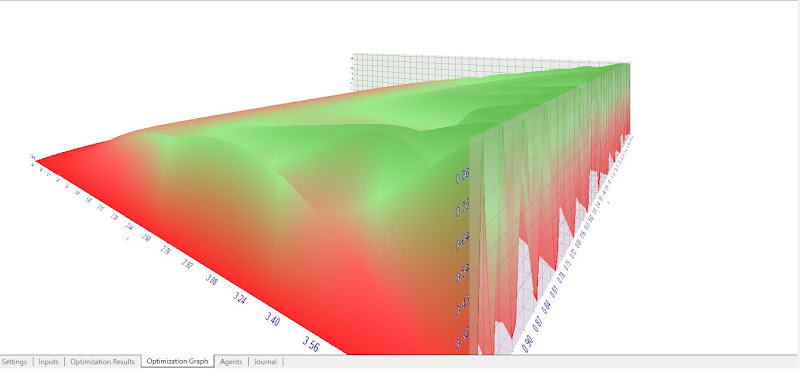От Поле за пускане
• JohnLast 2872 days ago

This code actually plots the logistic map, but as you will see the two first numbers are missing: first and second:

#property version "1.00"
//--- input parameters
input double x;
input int limitmap =10;
input double r=1;

//+------------------------------------------------------------------+
//| Tester function |
//+------------------------------------------------------------------+

double OnTester()
{
//---

double i=1,first=(r*x*(1-x)),second=r*first*(1-first);
double current;
do
{
current=r*second*(1-second);

first=second;
second=current;
i++; // without this operator an infinite loop will appear!
}
while(i<limitmap && !IsStopped());

//---
return(current);
}

Here is the Logistic map in the Metatrader 5 tester.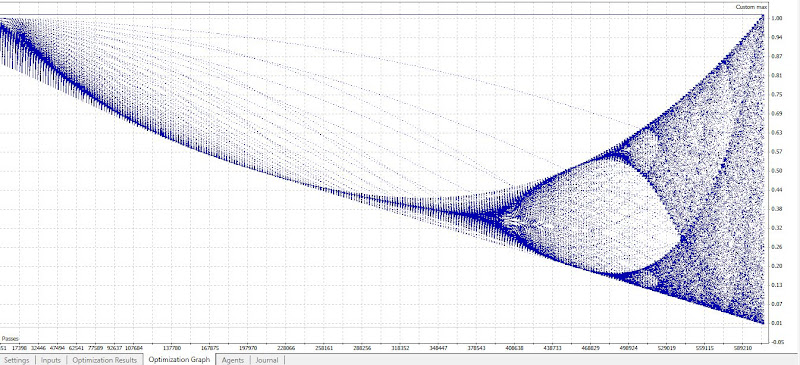От Поле за пускане
• JohnLast 2872 days ago

So the idea as as follows:

Given the logistic map equation:we can vary three parameters and to use this as a kernel:

1. We can vary x (from 0 to 1 with step 0.01, or 0.001 for example): this is giving us the initial conditions

2. We can vary the r parameter (from 1 to  with step 0.01, or 0.001 for example) this is the parameter governing the chaos.  r approximately 3.57 is the onset of chaos, so we can limit from 1 to 3.

3. And of course finally we can vary the limitmap parameter governing the number of iterations. This is the third parameter for the difference equation. Here I need some help because the code I made is missing to plot the values of first two iterations and it is beginning to plot from the third.

So We can call this chaos kernel, in fact I do not know even if that has a name. I did not find in the available litterature anything of this kind. So it is possible that this appears on this site for the first time. I know crazy it is.

The two MAIN ideas ARE:

- TO USE RECURRENT DIFFERENCE EQUATION

- TO USE SOME PORTION OF CHAOS OF THE KERNEL ITSELF

If that is true with just one kernel we have something extremely powerfull. And even more, there are many other maps waiting to be exploited as kernels.

So this is opening a door, for a new paradigm for the machine learning.

I am using here the logistic map because it is something extremely simple but generating extremely complex mathematical behavior.

Using chaos kernel to harness the chaos itself. Why not after all?

• JohnLast 2872 days ago

Another possibility is to use string equations for building dynamic kernel structures, but this is not as simple as the difference equations.

The difference equations are computationally light.

• JohnLast 2822 days ago

Finally the best code by now is those proposed by vgc:

double chaoskernel()
{
//---
double w, j;

w=(r*x*(1-x));
for(j=0;j<=limitmap;j++)
{
w=(r*w*(1-w));
}

double p1 = iCustom(TimeFrame,Symbol(),"PFE",x5,true,5,0,0);// Those are the input
double p2 = iCustom(TimeFrame,Symbol(),"PFE",x6,true,5,0,0);
double p3 = iCustom(TimeFrame,Symbol(),"PFE",x7,true,5,0,0);
double p4 = iCustom(TimeFrame,Symbol(),"PFE",x8,true,5,0,0);

return(w* p1 + w* p2 + w*p3 + w* p4);// We multiply the input value by the weight
}

• JohnLast 2767 days ago

Sometimes we would need more than one weight.

• jaguar1637 2767 days ago

how to know when to need another weight ?

• JohnLast 2767 days ago

You can chech here

I would use this, in fact the stupid mistake comes from simple mathematics, whenever we multiply by the same ammount on the left we get the same result if on the right side we have 0.

You can verify this by manually replacing the weight by different constants that you will get the same results in this case.

However when we touch a single variable that affects everything like in the neural asctrend a single kernel is enough.